Prealgebra 2e

# 2.5Prime Factorization and the Least Common Multiple

Prealgebra 2e2.5 Prime Factorization and the Least Common Multiple

### Learning Objectives

By the end of this section, you will be able to:

• Find the prime factorization of a composite number
• Find the least common multiple (LCM) of two numbers

### Be Prepared 2.12

Before you get started, take this readiness quiz.

Is $810810$ divisible by $2,3,5,6,or10?2,3,5,6,or10?$
If you missed this problem, review Example 2.44.

### Be Prepared 2.13

Is $127127$ prime or composite?
If you missed this problem, review Example 2.47.

Write $2⋅2⋅2⋅22⋅2⋅2⋅2$ in exponential notation.
If you missed this problem, review Example 2.5.

### Find the Prime Factorization of a Composite Number

In the previous section, we found the factors of a number. Prime numbers have only two factors, the number $11$ and the prime number itself. Composite numbers have more than two factors, and every composite number can be written as a unique product of primes. This is called the prime factorization of a number. When we write the prime factorization of a number, we are rewriting the number as a product of primes. Finding the prime factorization of a composite number will help you later in this course.

### Prime Factorization

The prime factorization of a number is the product of prime numbers that equals the number.

### Manipulative Mathematics

Doing the Manipulative Mathematics activity “Prime Numbers” will help you develop a better sense of prime numbers.

You may want to refer to the following list of prime numbers less than $5050$ as you work through this section.

$2,3,5,7,11,13,17,19,23,29,31,37,41,43,472,3,5,7,11,13,17,19,23,29,31,37,41,43,47$

#### Prime Factorization Using the Factor Tree Method

One way to find the prime factorization of a number is to make a factor tree. We start by writing the number, and then writing it as the product of two factors. We write the factors below the number and connect them to the number with a small line segment—a “branch” of the factor tree.

If a factor is prime, we circle it (like a bud on a tree), and do not factor that “branch” any further. If a factor is not prime, we repeat this process, writing it as the product of two factors and adding new branches to the tree.

We continue until all the branches end with a prime. When the factor tree is complete, the circled primes give us the prime factorization.

For example, let’s find the prime factorization of $36.36.$ We can start with any factor pair such as $33$ and $12.12.$ We write $33$ and $1212$ below $3636$ with branches connecting them.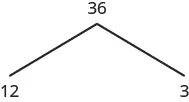The factor $33$ is prime, so we circle it. The factor $1212$ is composite, so we need to find its factors. Let’s use $33$ and $4.4.$ We write these factors on the tree under the $12.12.$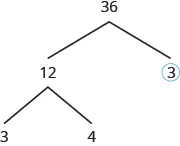The factor $33$ is prime, so we circle it. The factor $44$ is composite, and it factors into $2·2.2·2.$ We write these factors under the $4.4.$ Since $22$ is prime, we circle both $2s.2s.$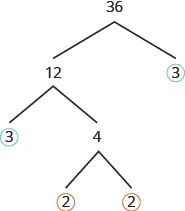The prime factorization is the product of the circled primes. We generally write the prime factorization in order from least to greatest.

$2⋅2⋅3⋅32⋅2⋅3⋅3$

In cases like this, where some of the prime factors are repeated, we can write prime factorization in exponential form.

$2⋅2⋅3⋅322⋅322⋅2⋅3⋅322⋅32$

Note that we could have started our factor tree with any factor pair of $36.36.$ We chose $1212$ and $3,3,$ but the same result would have been the same if we had started with $22$ and $18,418,4$ and $9,or6and6.9,or6and6.$

### How To

#### Find the prime factorization of a composite number using the tree method.

1. Step 1. Find any factor pair of the given number, and use these numbers to create two branches.
2. Step 2. If a factor is prime, that branch is complete. Circle the prime.
3. Step 3. If a factor is not prime, write it as the product of a factor pair and continue the process.
4. Step 4. Write the composite number as the product of all the circled primes.

### Example 2.48

Find the prime factorization of $4848$ using the factor tree method.

### Try It 2.95

Find the prime factorization using the factor tree method: $8080$

### Try It 2.96

Find the prime factorization using the factor tree method: $6060$

### Example 2.49

Find the prime factorization of 84 using the factor tree method.

### Try It 2.97

Find the prime factorization using the factor tree method: $126126$

### Try It 2.98

Find the prime factorization using the factor tree method: $294294$

#### Prime Factorization Using the Ladder Method

The ladder method is another way to find the prime factors of a composite number. It leads to the same result as the factor tree method. Some people prefer the ladder method to the factor tree method, and vice versa.

To begin building the “ladder,” divide the given number by its smallest prime factor. For example, to start the ladder for $36,36,$ we divide $3636$ by $2,2,$ the smallest prime factor of $36.36.$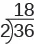To add a “step” to the ladder, we continue dividing by the same prime until it no longer divides evenly.Then we divide by the next prime; so we divide $99$ by $3.3.$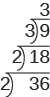We continue dividing up the ladder in this way until the quotient is prime. Since the quotient, $3,3,$ is prime, we stop here.

Do you see why the ladder method is sometimes called stacked division?

The prime factorization is the product of all the primes on the sides and top of the ladder.

$2⋅2⋅3⋅322⋅322⋅2⋅3⋅322⋅32$

Notice that the result is the same as we obtained with the factor tree method.

### How To

#### Find the prime factorization of a composite number using the ladder method.

1. Step 1. Divide the number by the smallest prime.
2. Step 2. Continue dividing by that prime until it no longer divides evenly.
3. Step 3. Divide by the next prime until it no longer divides evenly.
4. Step 4. Continue until the quotient is a prime.
5. Step 5. Write the composite number as the product of all the primes on the sides and top of the ladder.

### Example 2.50

Find the prime factorization of $120120$ using the ladder method.

### Try It 2.99

Find the prime factorization using the ladder method: $8080$

### Try It 2.100

Find the prime factorization using the ladder method: $6060$

### Example 2.51

Find the prime factorization of $4848$ using the ladder method.

### Try It 2.101

Find the prime factorization using the ladder method. $126126$

### Try It 2.102

Find the prime factorization using the ladder method. $294294$

### Find the Least Common Multiple (LCM) of Two Numbers

One of the reasons we look at multiples and primes is to use these techniques to find the least common multiple of two numbers. This will be useful when we add and subtract fractions with different denominators.

#### Listing Multiples Method

A common multiple of two numbers is a number that is a multiple of both numbers. Suppose we want to find common multiples of $1010$ and $25.25.$ We can list the first several multiples of each number. Then we look for multiples that are common to both lists—these are the common multiples.

$10:10,20,30,40,50,60,70,80,90,100,110,… 25:25,50,75,100,125,… 10:10,20,30,40,50,60,70,80,90,100,110,… 25:25,50,75,100,125,…$

We see that $5050$ and $100100$ appear in both lists. They are common multiples of $1010$ and $25.25.$ We would find more common multiples if we continued the list of multiples for each.

The smallest number that is a multiple of two numbers is called the least common multiple (LCM). So the least LCM of $1010$ and $2525$ is $50.50.$

### How To

#### Find the least common multiple (LCM) of two numbers by listing multiples.

1. Step 1. List the first several multiples of each number.
2. Step 2. Look for multiples common to both lists. If there are no common multiples in the lists, write out additional multiples for each number.
3. Step 3. Look for the smallest number that is common to both lists.
4. Step 4. This number is the LCM.

### Example 2.52

Find the LCM of $1515$ and $2020$ by listing multiples.

### Try It 2.103

Find the least common multiple (LCM) of the given numbers: $9and129and12$

### Try It 2.104

Find the least common multiple (LCM) of the given numbers: $18and2418and24$

#### Prime Factors Method

Another way to find the least common multiple of two numbers is to use their prime factors. We’ll use this method to find the LCM of $1212$ and $18.18.$

We start by finding the prime factorization of each number.

$12=2⋅2⋅318=2⋅3⋅312=2⋅2⋅318=2⋅3⋅3$

Then we write each number as a product of primes, matching primes vertically when possible.

$12=2⋅2⋅3 18=2⋅3⋅312=2⋅2⋅3 18=2⋅3⋅3$

Now we bring down the primes in each column. The LCM is the product of these factors.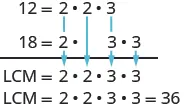Notice that the prime factors of $1212$ and the prime factors of $1818$ are included in the LCM. By matching up the common primes, each common prime factor is used only once. This ensures that $3636$ is the least common multiple.

### How To

#### Find the LCM using the prime factors method.

1. Step 1. Find the prime factorization of each number.
2. Step 2. Write each number as a product of primes, matching primes vertically when possible.
3. Step 3. Bring down the primes in each column.
4. Step 4. Multiply the factors to get the LCM.

### Example 2.53

Find the LCM of $1515$ and $1818$ using the prime factors method.

### Try It 2.105

Find the LCM using the prime factors method. $15and2015and20$

### Try It 2.106

Find the LCM using the prime factors method. $15and3515and35$

### Example 2.54

Find the LCM of $5050$ and $100100$ using the prime factors method.

### Try It 2.107

Find the LCM using the prime factors method: $55,8855,88$

### Try It 2.108

Find the LCM using the prime factors method: $60,7260,72$

### Section 2.5 Exercises

#### Practice Makes Perfect

Find the Prime Factorization of a Composite Number

In the following exercises, find the prime factorization of each number using the factor tree method.

267.

$86 86$

268.

$78 78$

269.

$132 132$

270.

$455 455$

271.

$693 693$

272.

$420 420$

273.

$115 115$

274.

$225 225$

275.

$2475 2475$

276.

1560

In the following exercises, find the prime factorization of each number using the ladder method.

277.

$56 56$

278.

$72 72$

279.

$168 168$

280.

$252 252$

281.

$391 391$

282.

$400 400$

283.

$432 432$

284.

$627 627$

285.

$2160 2160$

286.

$2520 2520$

In the following exercises, find the prime factorization of each number using any method.

287.

$150 150$

288.

$180 180$

289.

$525 525$

290.

$444 444$

291.

$36 36$

292.

$50 50$

293.

$350 350$

294.

$144 144$

Find the Least Common Multiple (LCM) of Two Numbers

In the following exercises, find the least common multiple (LCM) by listing multiples.

295.

$8 , 12 8 , 12$

296.

$4 , 3 4 , 3$

297.

$6 , 15 6 , 15$

298.

$12 , 16 12 , 16$

299.

$30 , 40 30 , 40$

300.

$20 , 30 20 , 30$

301.

$60 , 75 60 , 75$

302.

$44 , 55 44 , 55$

In the following exercises, find the least common multiple (LCM) by using the prime factors method.

303.

$8 , 12 8 , 12$

304.

$12 , 16 12 , 16$

305.

$24 , 30 24 , 30$

306.

$28 , 40 28 , 40$

307.

$70 , 84 70 , 84$

308.

$84 , 90 84 , 90$

In the following exercises, find the least common multiple (LCM) using any method.

309.

$6 , 21 6 , 21$

310.

$9 , 15 9 , 15$

311.

$24 , 30 24 , 30$

312.

$32 , 40 32 , 40$

#### Everyday Math

313.

Grocery shopping Hot dogs are sold in packages of ten, but hot dog buns come in packs of eight. What is the smallest number of hot dogs and buns that can be purchased if you want to have the same number of hot dogs and buns? (Hint: it is the LCM!)

314.

Grocery shopping Paper plates are sold in packages of $1212$ and party cups come in packs of $8.8.$ What is the smallest number of plates and cups you can purchase if you want to have the same number of each? (Hint: it is the LCM!)

#### Writing Exercises

315.

Do you prefer to find the prime factorization of a composite number by using the factor tree method or the ladder method? Why?

316.

Do you prefer to find the LCM by listing multiples or by using the prime factors method? Why?

#### Self Check

After completing the exercises, use this checklist to evaluate your mastery of the objectives of this section.

Overall, after looking at the checklist, do you think you are well-prepared for the next Chapter? Why or why not?

Order a print copy

As an Amazon Associate we earn from qualifying purchases.